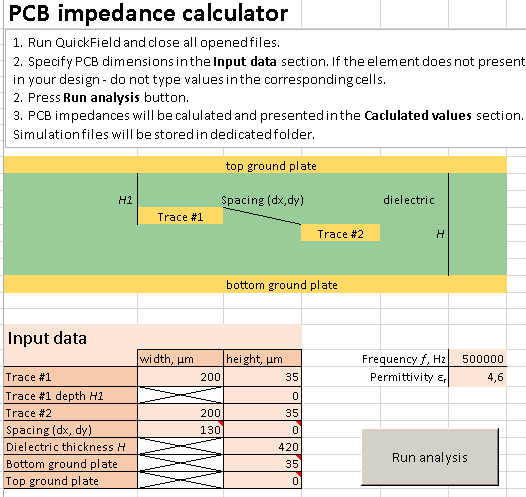# PCB impedance calculator

free tool for QuickField simulation software, microstrip impedance calculatorThis script calculates the impedance of the printed circuit board with two conducting traces positioned in the dielectric layer between two ground plates.
Conductors have rectangular cross-section with user defined width and height. Position of the first conductor is defined by the distance from the top grounded plate. Position of the second conductor is defined by X and Y spacing from the first conductor. The signal frequency and dielectric permittivity are user-defined as well.
Both electrostatic and magnetostatic analyses are performed for the PCB cross-section for self and mutual capacitances (C0, Cdiff) and inductances (L0, Ldiff) calculations. Corresponding FEA problems are automatically created and analyzed using QuickField API ActiveField